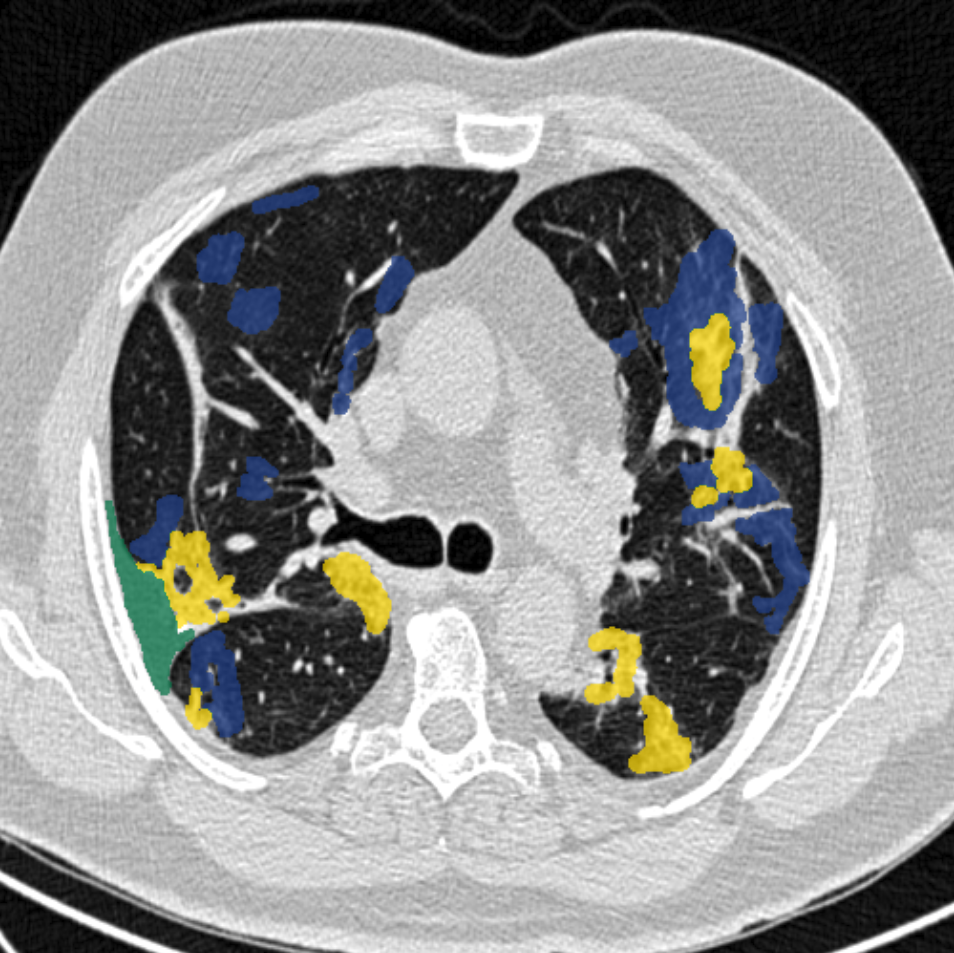# Covid-19 radiology — data collection and preparation for Artificial Intelligence

Original article can be found here (source): Artificial Intelligence on MediumAll coding was done in Python. The resulting NIFTI-files, both normalized images, and masks are openly available at medicalsegmenation.com/covid19. Further, we used this data to train a U-Net model, which is uploaded and available interactively in MedSeg, through TensorflowJS. We do not describe this last part as this is well documented by many others.

Complete code:

Step 1: Import relevant libraries

`import numpy as npimport osimport nibabel as nibimport matplotlib.image as mpimgfrom skimage.transform import resize`

Step 2: Count your unique jpg-images in your relevant folder (in case some other files have snuck in)

`counter = 0for i in os.listdir("JPG_directory/"): if i.endswith(".jpg"): counter+=1print(counter)`

Step 3: Create a new array that will become a NIFTI-file. The shape is of 512 x 512 (pixel size) and counter (the number of JPGs you want to include).

`new_nifti = np.zeros((512, 512, counter))`

Step 4: Add the jpg-images to your array by resizing them to 512 x 512, grayscaling and finally flipping them, so that the orientation is correct when saved as NIFTI.

`counter = 0for i in os.listdir("JPG_directory/"): if i.endswith(".jpg"): img = mpimg.imread("JPG_directory/"+i) img = img.astype("float64") resized_img = resize(img, (512,512,3), preserve_range=True) resized_img = resized_img[:,:,0] fl_resized_img = np.fliplr(np.rot90(resized_img, k=3)) new_nifti[:,:,counter] = fl_resized_img counter+=1`

Step 5: Save your new NIFTI-file containing the resized JPG COVID-19 images

`savefile = nib.Nifti1Image(new_nifti, None)nib.save(savefile, "JPG_nifti.nii")`

Step 6: Time to normalize the images. We create a normalize function, here we use fat as -100 HU and air as -1000 HU:

`def normalize_function(img, air, fat): air_HU = -1000 fat_HU = -100 delta_air_fat_HU = abs(air_HU - fat_HU) delta_air = abs(air - air_HU) delta_fat_air_rgb = abs(fat - air) ratio = delta_air_fat_HU / delta_fat_air_rgb img = img - air img = img * ratio img = img + air_HU return img`

Step 7: Obtain the unique intensity values for each image denoting air and fat. We used our own tool on MedSeg to establish an average using an ROI and added two mask-labels to each image, one for fat and one for air (see example above). Save this mask file and load it along with the original NIFTI-file containing the images.

`mask = nib.load("MASK_FILE_WITH_FAT_AIR_LABELS.nii")mask_np = np.array(mask.get_fdata())rgb_image = nib.load("JPG_nifti.nii")rgb_image_np = np.array(rgb_image.get_fdata())`

Step 8: In case you have chosen not to use all of the images you have compiled, it is best to sort the useless ones out

`counter = 0for i in range(mask_np.shape): if len(np.unique(mask_np[:,:,i]))==3: counter+=1print(counter)new_normalized_nifti = np.zeros((512, 512, counter))`

Step 9: Use the normalizing function to prepare the images into one NIFTI-file ready for segmentation

`counter = 0for i in range(mask_np.shape): unique_values = np.unique(mask_np[:,:,i]) if len(unique_values)==3: air = unique_values fat = unique_values rgb_slice = rgb_image_np[:,:,i] normalized_slice = normalize_function(rgb_slice, air, fat) new_normalized_nifti[:,:,counter] = normalized_slice counter+=1`

Step 10: Save your new NIFTI-file containing all the converted JPGs which are now normalized

`savefile = nib.Nifti1Image(new_normalized_nifti, None)nib.save(savefile, "COVID-19.nii")`